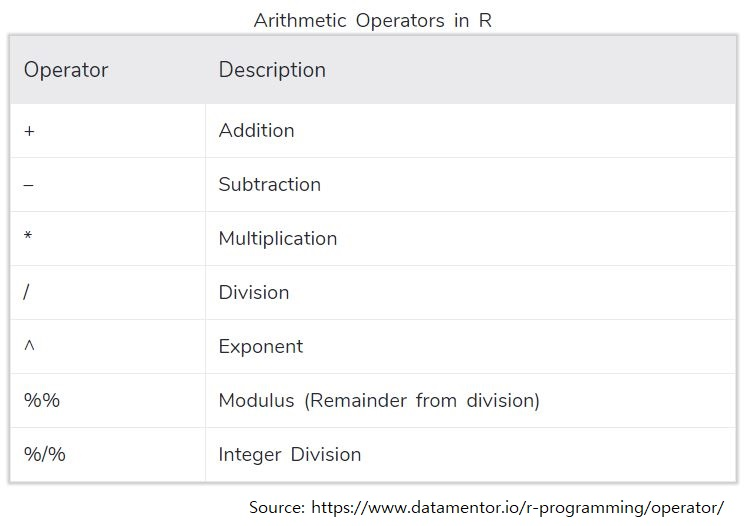## Arithmetic operators

These are operators for math calculations.Examples below show simple calculations (mainly) using the arithmetic operators.

``4+10   ``
``##  14``
``5+(2*3)``
``##  11``
``3^2``
``##  9``
``sqrt(3^2)   # Square root of 3^2``
``##  3``
``````a <- 6 ; b <- 10*3
b/a``````
``##  5``